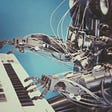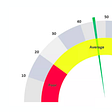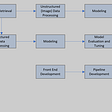# K-Nearest Neighbors (KNN)

In this Blog I will be writing about a very famous supervised learning algorithm, that is, k-nearest neighbors or in short KNN.

Here I will explain about what is KNN algorithm, Industrial uses of KNN algorithm, How the KNN algorithm works, How to choose the value of K, Advantages/Disadvantages and finally I will provide link to my briefly explained Jupyter Notebook on implementation of KNN algorithm.

Also I will provide link to my Digit classification (on mnsit dataset) implemented using KNN algorithm. So without any further due lets get started.

# What is KNN algorithm?

KNN is a model that classifies data points based on the points that are most similar to it. It uses test data to make an “educated guess” on what an unclassified point should be classified as.

KNN is an algorithm that is considered both non-parametric and an example of lazy learning. What do these two terms mean exactly?

• Non-parametric means that it makes no assumptions. The model is made up entirely from the data given to it rather than assuming its structure is normal.
• Lazy learning means that the algorithm makes no generalizations. This means that there is little training involved when using this method. Because of this, all of the training data is also used in testing when using KNN.

What is K in KNN?

k = Number of nearest neighbor

If k=1, then test examples are given the same label as the closest example in the training set. If k=3, the labels of the three closest classes are checked and the most common (i.e., occurring at least twice) label is assigned, and so on for larger k’s.

When you build a k-nearest neighbor classifier, you choose the value of k. You might have a specific value of k in mind, or you could divide up your data and use something like cross-validation to test several values of k in order to determine which works best for your data. For n=1000 cases, I would bet that the optimal k is somewhere between 1 and 19, but you’d really have to try it to be sure.

# Industrial Application of KNN Algorithm

K-nearest algorithm is used in various sectors of day to day life. It is easy to use so that data scientists and the beginner of machine learning use this algorithm for a simple task. Some of the uses of the k nearest neighbor algorithm are:

## Finding diabetics ratio

Diabetes diseases are based on age, health condition, family tradition, and food habits. But is a particular locality we can judge the ratio of diabetes based on the K Nearest Neighbor Algorithm. If you figure out the data of is age, pregnancies, glucose, blood pressure, skin thickness, insulin, body mass index and other required data we can easily plot the probability of diabetes at a certain age.

## Recommendation System

If we search any product to any online store it will show the product. Decide that particular product it recommends some other product. You will be astonished after knowing that the 35% revenue of Amazon comes from the recommendation system. Decide the online store, YouTube, Netflix, and all search engines use the algorithms of k-nearest neighbor.

## Concept Search

Concept search is the industrial application of the K Nearest Neighbor Algorithm. It means searching for similar documents simultaneously. The data on the internet is increasing every single second. The main problem is extracting concepts from the large set of databases. K-nearest neighbor helps to find the concept from the simple approach.

## Finding The Ratio of Breast Cancer

In the medical sector, the KNN algorithm is widely used. It is used to predict breast cancer. Here KNN algorithm is used as the classifier. The K nearest neighbor is the easiest algorithm to apply here. Based on the previous history of the locality, age and other conditions KNN is suitable for labeled data.

# How the KNN Algorithm Works

Consider a dataset having two variables weight and height. Each of the points is classified as overweight, normal and underweight. No I giving a data set:

Now if I give the value of 157 cm height. The data is not given previously. Based on the nearest value it predicts the weight of 157 cm. It is using the model of k nearest neighbor.

We can implement the k nearest neighbor algorithm by using Euclidean distance formula. It works by determining the distance of two coordinates. In a graph, if we plot the value of (x,y) and (a,b) then we will imply the formula as:

dist(d) = ✓(x-a)² + (y-b)²

# Choosing the right value for K

To select the K that’s right for your data, we run the KNN algorithm several times with different values of K and choose the K that reduces the number of errors we encounter while maintaining the algorithm’s ability to accurately make predictions when it’s given data it hasn’t seen before.

Here are some things to keep in mind:

1. As we decrease the value of K to 1, our predictions become less stable. Just think for a minute, imagine K=1 and we have a query point surrounded by several reds and one green (I’m thinking about the top left corner of the colored plot above), but the green is the single nearest neighbor. Reasonably, we would think the query point is most likely red, but because K=1, KNN incorrectly predicts that the query point is green.
2. Inversely, as we increase the value of K, our predictions become more stable due to majority voting / averaging, and thus, more likely to make more accurate predictions (up to a certain point). Eventually, we begin to witness an increasing number of errors. It is at this point we know we have pushed the value of K too far.
3. In cases where we are taking a majority vote (e.g. picking the mode in a classification problem) among labels, we usually make K an odd number to have a tiebreaker.

1. The algorithm is simple and easy to implement.
2. There’s no need to build a model, tune several parameters, or make additional assumptions.
3. The algorithm is versatile. It can be used for classification, regression, and search (as we will see in the next section).

1. The algorithm gets significantly slower as the number of examples and/or predictors/independent variables increase.

Here’s my Jupyter Notebook of a briefly explained implementation on KNN (From scratch).

Here’s my Jupyter Notebook of a briefly explained implementation of Digit classification on mnsit data using KNN (Using inbuilt model).

I tried to provide all the important information on getting started with Linear Regression and its implementation. I hope you will find something useful here. Thank you for reading till the end.

--

--

--

## More from Analytics Vidhya

Analytics Vidhya is a community of Analytics and Data Science professionals. We are building the next-gen data science ecosystem https://www.analyticsvidhya.com

## Curriculum Learning and Symbolic Mathematics## Increasing The Accuracy Of a Deep Learning Model Using DevOps## Can Privacy and Machine Learning Work Together?## How To: Deploy GPT2 NLG with Flask on AWS ElasticBeanstalk## Tensorflow Performance on the new M1 Max MacBook## Contrastive Learning in Computer Vision —  brief concept overview and sample code.## Image Segmentation with K-Means Clustering## Predicting Patient Adopters Through Deep Learning## Shubhang Agrawal

Tech Enthusiast, Gamer

## Decision Tree and Random Forest from Scratch## The Math Behind the K-means and Hierarchical Clustering Algorithm!## Evaluating classification models with Accuracy, Precision and Recall.